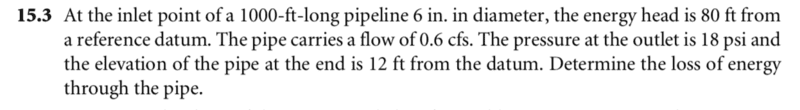# Hydraulics help please -- Energy loss in a long pipeline

## Homework Statement## Homework Equations

y1 + P1/##\gamma##+v1/2g=y2 + P2/##\gamma##+v2/2g+hL

## The Attempt at a Solution

I used the above equation and assumed that P1=0 and both velocity heads are equal. Is that valid? However I did not need to use the geometry of the pipe so I think I am doing something wrong. Can someone point me in the right direction?

#### Attachments

Last edited by a moderator:

I think you are right, the pipe is uniform, but since the problem asked for energy loss, you must derive (from the energy conservation equation) a correct equation that has some kind of energy loss. In the end it must be some kind of power loss ##P=\frac {dW} {dt}##.
Sorry I loss my mind for a moment, yes it's the loss per feet.

Last edited:
Im looking for energy loss in terms of feet not power loss. I have a lot of information
Pressure
heights
flow rate
length
diameter
no roughness
what is throwing me off is that if I use general energy equation are my assumptions correct that P1=0 and that velocity heads are eqaual?
If i use darcy weisbach eq. i do not have temperature or roughness to help me determine friction factor

I think P1 is 1atm, not 0 like you assumed, this pressure (P1) only equal zero if the head is placed in vacuum, or the inlet pressure is the reference pressure. In the first case the water would be boiled off to vacuum and then this question would be invalid. In the second case I believe they must first say that the reference is at that point (the inlet). They just say the pressure at the outlet is 18psi so that mean it is the absolute pressure (reference to vacuum) and that the inlet pressure is at normal condition of 1atm. For the velocity I think they are equal.

Chestermiller
Mentor
What is the definition of "energy head?"

potential energy of a static column of water (height) + velocity head + pressure head... the equation I have written above

Chestermiller
Mentor
The give you the energy head at point 1 and they give you everything you need to calculate the energy head at point 2. The difference is the energy loss (head).

So my assumptions are correct? velocity heads are the same and that pressure at the top = 0? And while I have you here, does Darcy's equation and Heinz-Williams yield the same loss? can I use them in combination to solve for a particular problem?

Chestermiller
Mentor
So my assumptions are correct? velocity heads are the same and that pressure at the top = 0? And while I have you here, does Darcy's equation and Heinz-Williams yield the same loss? can I use them in combination to solve for a particular problem?
None of this is right. We have $$y_1+\frac{p_1}{\gamma}+\frac{v_1^2}{2g}=80\ ft$$From the information provided in the problem statement, what are ##y_2##, ##\frac{P_2}{\gamma}##, and ##\frac{v_2^2}{2g}##?

y2 = 12
P2/##\gamma## = 18*144/62.4 =41.5
velocity head @ section 2 = (Q/A)2/64.4 = .14

solving for hL = 80 - 12 - 41.5 - .14 = 26.36 ft

why is P1 = 0? and why is velocity head @ section 1 = 0?

Chestermiller
Mentor
y2 = 12
P2/##\gamma## = 18*144/62.4 =41.5
velocity head @ section 2 = (Q/A)2/64.4 = .14

solving for hL = 80 - 12 - 41.5 - .14 = 26.36 ft
Correct
why is P1 = 0? and why is velocity head @ section 1 = 0?
Who says?[/QUOTE]

OOOOOHHHH I see, problem states energy head for section 1 as a whole is 80 ft I was fixated that 80ft was y1... got it

Thank you.

On another note whenever I am solving a problem with a two reservoir system we assume velocity heads are zero because of their size the dh/dt is basically 0 correct? So if I have a system with only one reservoir i will have an equation like so, Energy head section 1 = velocity head + hL can I use continuity equation to solve for velocity or will velocity vary with head loss?

Chestermiller
Mentor
OOOOOHHHH I see, problem states energy head for section 1 as a whole is 80 ft I was fixated that 80ft was y1... got it

Thank you.

On another note whenever I am solving a problem with a two reservoir system we assume velocity heads are zero because of their size the dh/dt is basically 0 correct? So if I have a system with only one reservoir i will have an equation like so, Energy head section 1 = velocity head + hL can I use continuity equation to solve for velocity or will velocity vary with head loss?
For any reservoir, dh/dt is usually small.

•roam